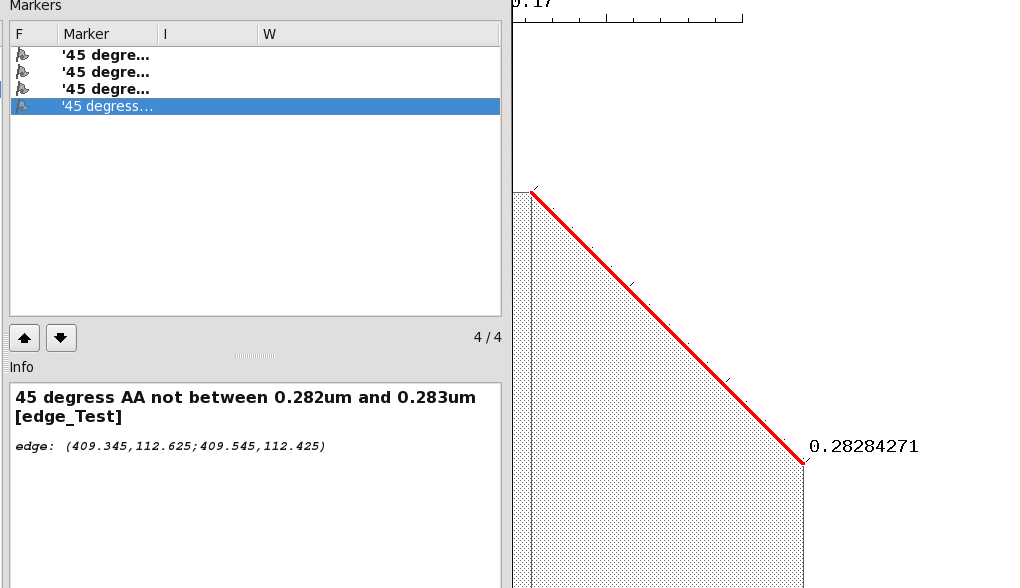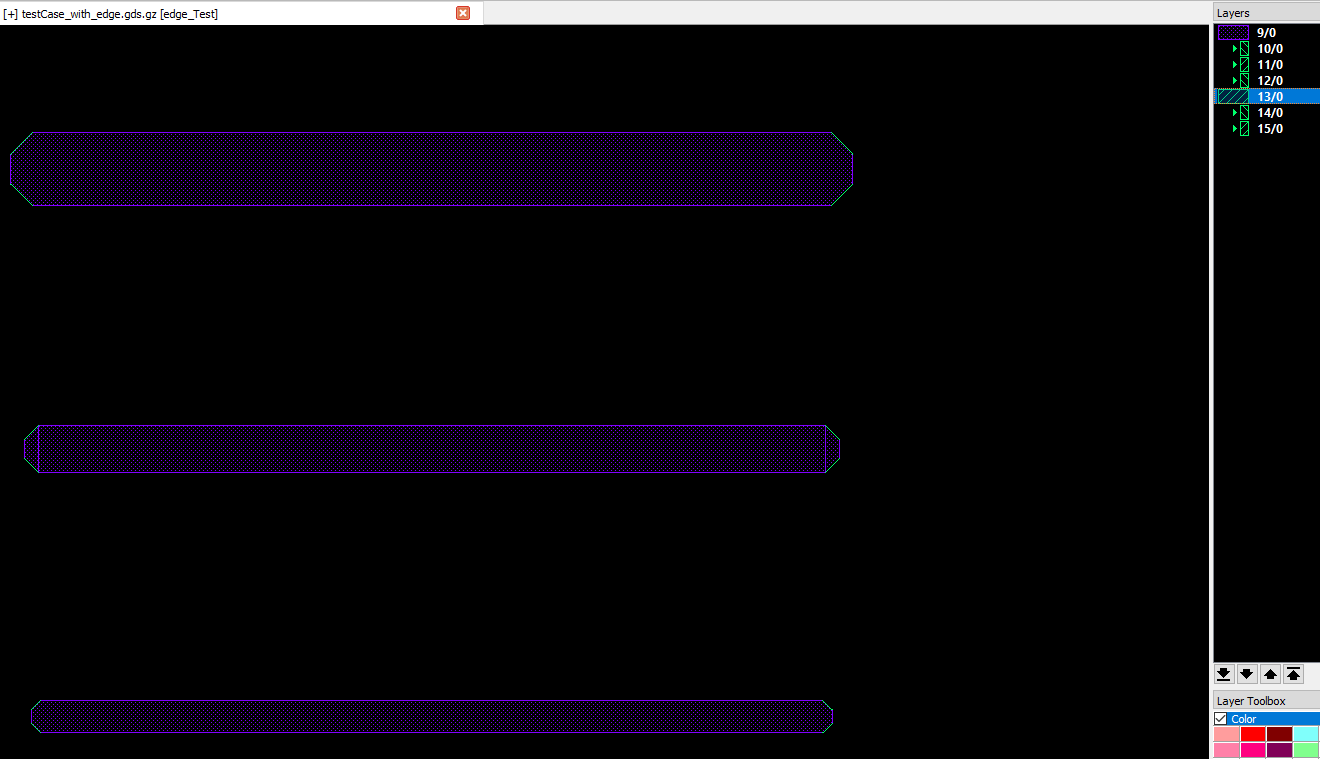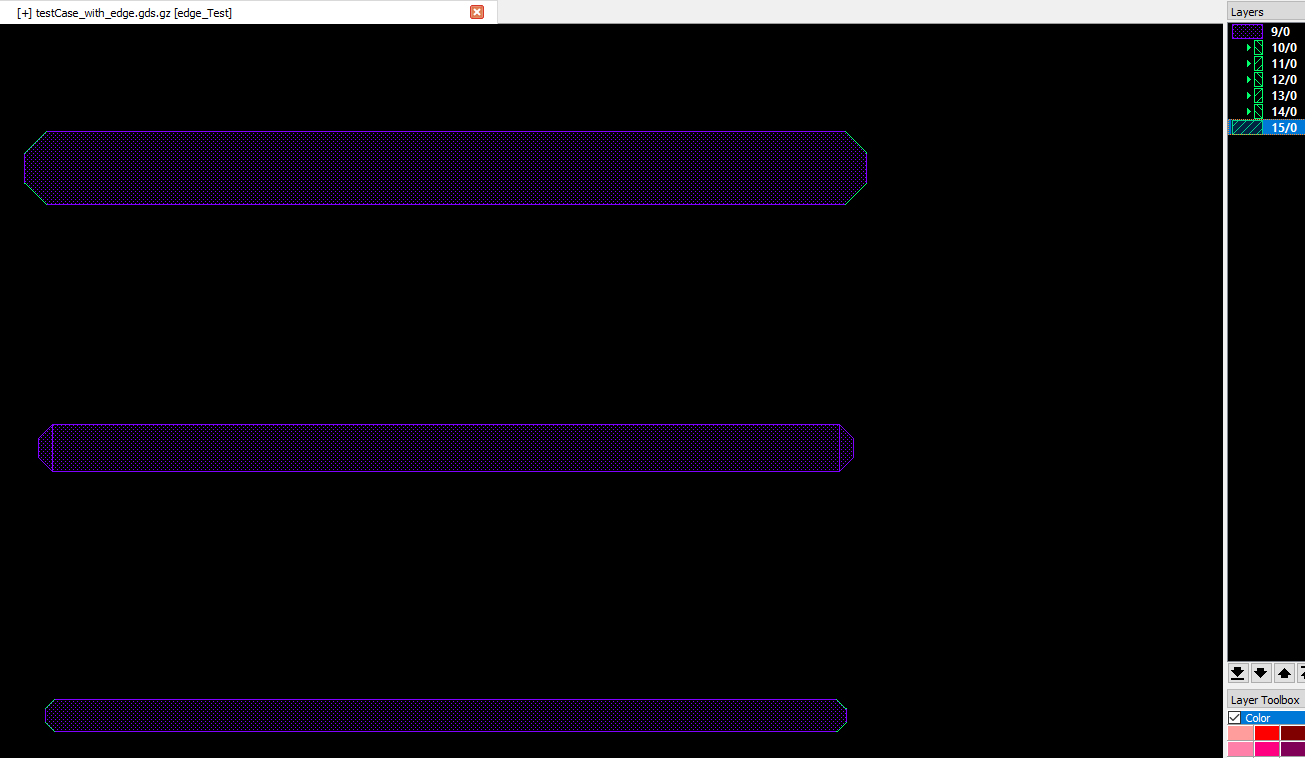# Is there a bug in with_length/without_length DRC method?

edited June 2021

Hi!
I have written a short DRC script to check that 45 degrees chopping was done correctly.
The DRC script collects the 45 (and 135) degrees edges, and then checks that they are indeed in the range of 0.282 and 0.283um.
Any edge outside of this range should trigger a violation.

``````report ("testing")
ACTIVE=input(9,0)
AA_45=(ACTIVE.edges.with_angle(45.degree) + ACTIVE.edges.with_angle(135.degree))
AA_45.without_length(0.282.um,0.283.um).output("45 degrees AA not between 0.282um and 0.283um")
``````

Strangely, the DRC alerts of all polygons that are ~0.2828um.Am I missing anything?
PS: also attached a GDS test case. I am using Klayout version 0.26.3
Thanks!
Allon

• Hi Allon,

I tried some things: dd_45 and ee_45 don't work, but ff_45 seems to work. It looks like the length is rounded to the nearest DBU?

Cheers,

Tomas

``````active=input(9,0)

aa_45= active.edges.with_angle(45.degree) + active.edges.with_angle(135.degree)

aa_45.output(10,0)

bb_45 = aa_45.without_length(0.28 .. 0.285)

cc_45 = aa_45.without_length(0.281 .. 0.284)

dd_45 = aa_45.without_length(0.282 .. 0.283)

ee_45 = aa_45.without_length(0.283 .. 0.283)

ff_45 = aa_45.without_length(0.283)

bb_45.output(11,0)

cc_45.output(12,0)

dd_45.output(13,0)

ee_45.output(14,0)

ff_45.output(15,0)
``````• @allonpa If you check `without_length(0.282.um,0.283.um)` this will actually mean "excluding 0.283". But the lengths are internally rounded to DBU before compare, so the measure length will be 0.283 and that (because of the "excluding" definition) will fall into the "without_length" range.

So to waive everything between 0.282 and 0.283 you'll actually need `without_length(0.282.um,0.284.um)`. Strictly speaking however this will waive lengths between 0.2815 and 0.2835.

Matthias

• OK. Thanks @tomas2004, @Matthias. Fully understood, and works well.
Best regards, Allon.